## If you want to determine the actual salicylate concentration for a sample that gives a greater absorption than the highest concentration sta

Question

If you want to determine the actual salicylate concentration for a sample that gives a greater absorption than the highest concentration standard (as described in Question (3)), you need to dilute the sample. If you dilute it by mixing 0.25 mL of sample with 0.75 mL of water and then find that this mixture has a salicylate concentration of 0.45 mg/mL, what is the concentration of the unknown sample

in progress 0
5 months 2021-08-31T09:56:19+00:00 1 Answers 0 views 0

1. Answer: The concentration of unknown sample is 1.8 mg/ml

Explanation:

According to the dilution law,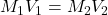where,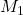= concentration of stock solution = ?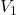= volume of stock solution = 0.25 ml= concentration of diluted solution = 0.45 mg/ml= volume of diluted solution = (0.25+0.75) ml = 1.00 ml

Putting in the values we get: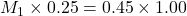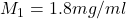Therefore, concentration of unknown sample is 1.8 mg/ml